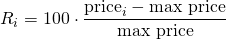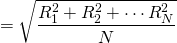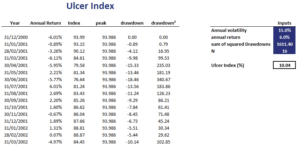# Ulcer index

The Ulcer Index indicator is a risk-measure proposed by Peter Martin (who also proposed the Martin ratio or Ulcer performance Index) that is used a lot in the context of technical analysis. The measure was first mentioned in 1987 in a book by Peter Martin and Byron McCann called “The Investors Guide to Fidelity funds”. The Ulcer index (UI) is a measure of downside volatility that is based on drawdowns. Thus the measure focuses on downside risk. The UI derives its name from the fact that stress from investment losses may be expected to lead to stomach ulcers.

## Ulcer Index formula

To calculate the Ulcer Index, we first calculate the following index R over N dayswhere maxprice is the highest price over the period of N days. Then, we take the quadratic mean of the values of the index Ri over time. This is similar to a calculation of the standard deviationThe calculation also implies that the index is always positive.

## Ulcer Index calculation

Finally, let’s illustrate the index calculation using a numerical example. In particular, suppose we have a time series of monthly returns. The following figure illustrates the calculation of the Ulcer Index.The spreadsheet that implements the Ulcer index calculation is available at the bottom of the page.

## Ulcer index usage

There are two main ways of using the index. First, we can use it in the context of technical analysis. In that case, we can calculate a moving average time series of the UI. We can then use it to analyze the amount of downside risk. Alternatively, we can use the measures to calculate a measure of risk-adjusted performance. In that case, we obtain the Martin ratio (sometimes also called the Ulcer performance index).

## Summary

We discussed the UI, a measure of downside risk that is commonly used by analysts that employ technical analysis. The UI is typically used to construct a risk-adjusted performance measure similar to the Sharpe ratio called the Ulcer performance index. Alternatively, the index can be used in the context of technical analysis.

### Calculator

Want to have an implementation in Excel? Download the Excel file: Ulcer Index template